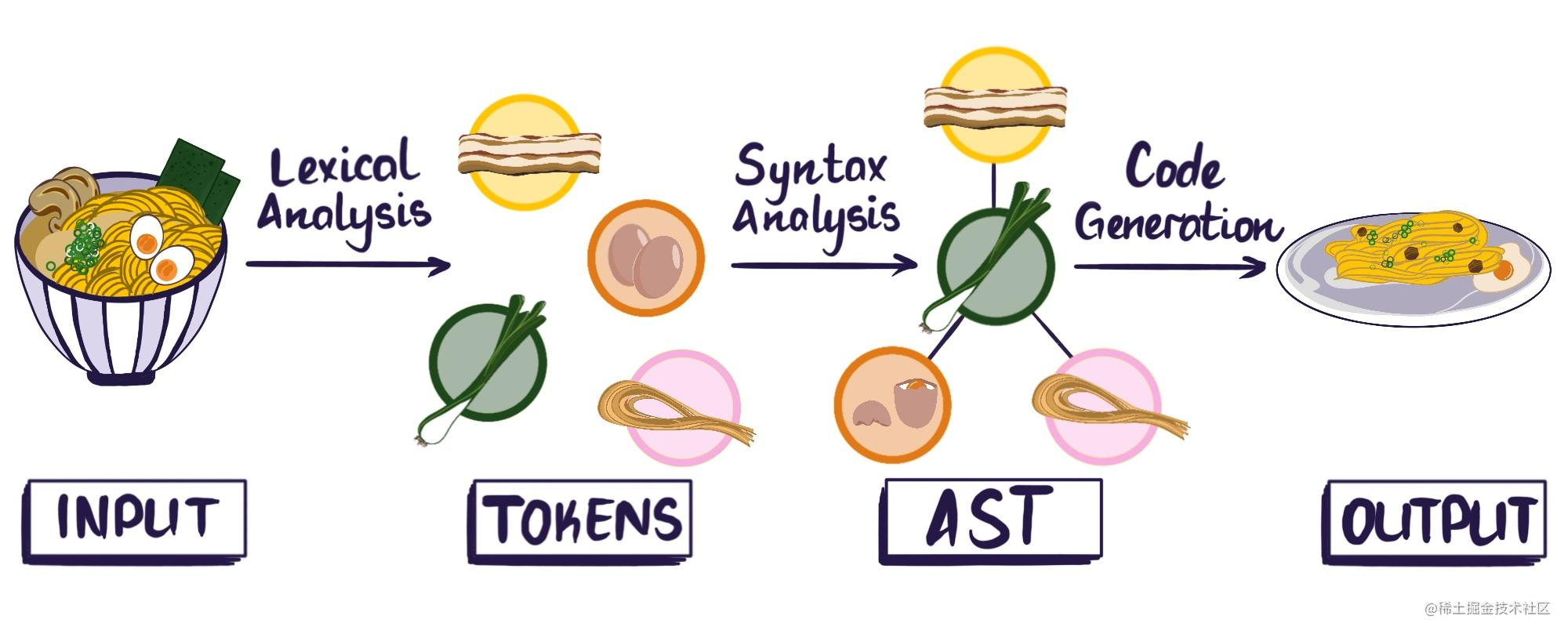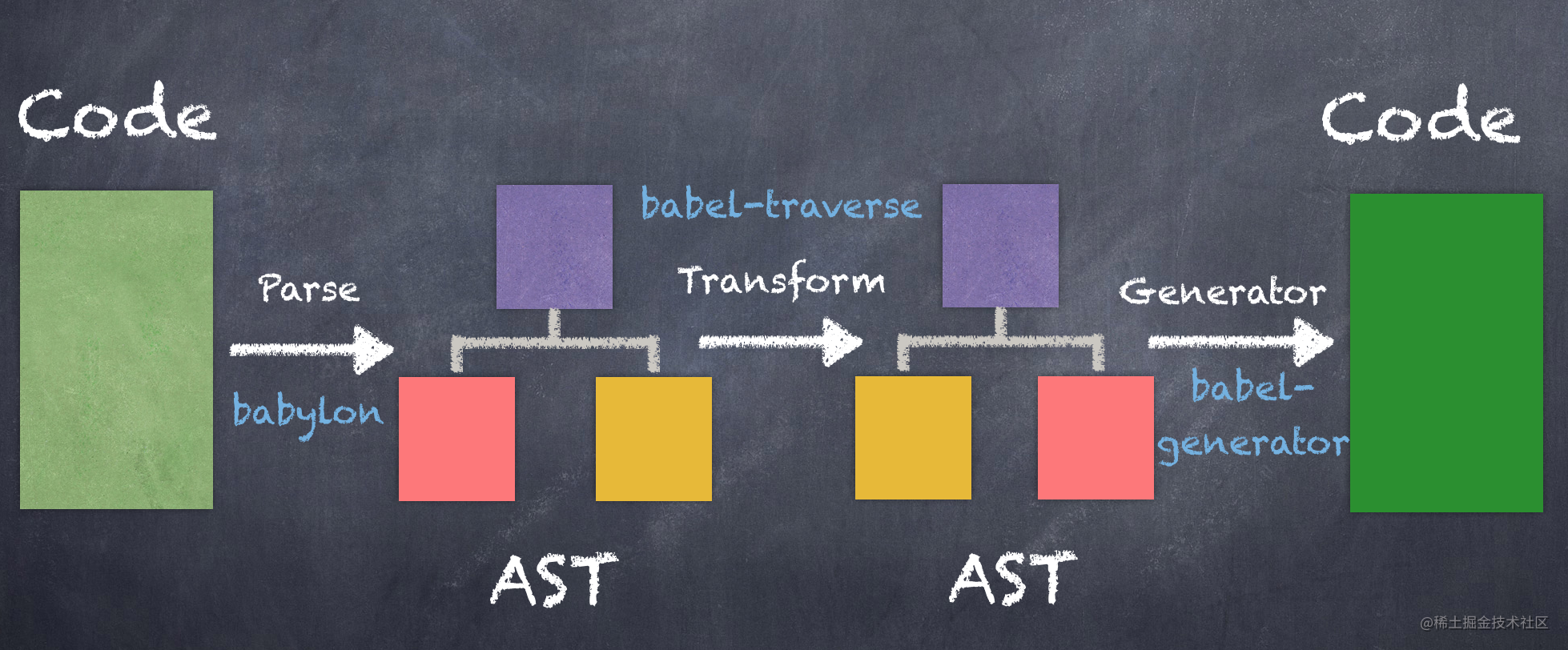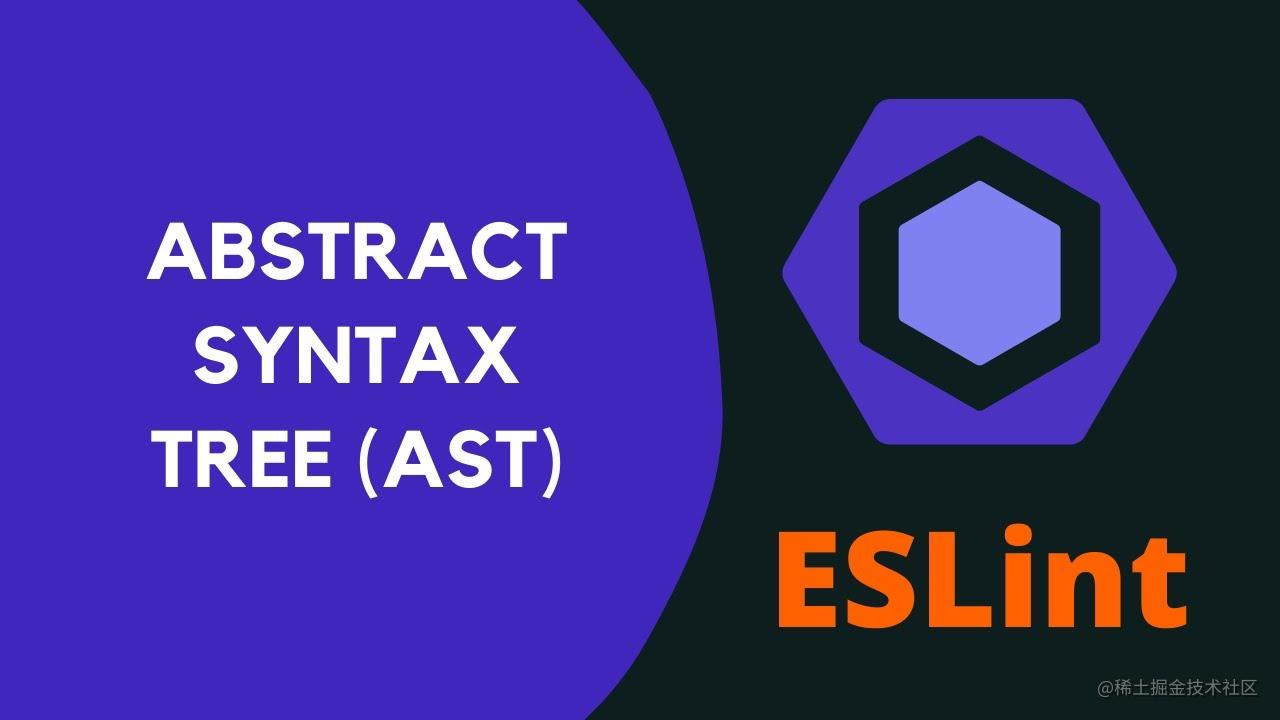# 动手写一个 javascript 代码解析器(1)

### 动机#### 什么是 ASTAST 是 Abstract Syntax Tree(抽象语法树)的缩写。

### 最佳实践#### ESLint

ESLint是一个用来检查和报告 JavaScript 编写规范的插件化工具，通过配置规则来规范代码，高质量 developer 写高质量 code(javascript 版) 这篇分享提到这个工具

#### Vue-cli

vue-cli 得都是依赖 AST 进行开发的

#### webpack

webpack源码解析 AST 并不是使用 acorn。webpack自己实现了一个JavascriptParser类，这个类里面用到了acorn。

### 解析器

``````const A = require('arcsecond');

const stringParser = A.str('hello');
const result = stringParser.run(
"hello"
)

console.log(result)//{ isError: false, result: 'hello', index: 5, data: null }

`A.str` 创建一个文本解析器，具体作用有点类似正则匹配，调用该方法是传入一个要匹配的文本，然后返回一个解析器用于提取该字符串，` stringParser.run( "hello")` 调用解析器 `run`方法，传入要在其中匹配字符串,后会返回一个对象。

• isError 表示是否解析到字符串
• result: 返回解析结构
• index: 返回解析后索引位置
• data: 数据
``````const stringParser = A.str("hello");

resstringParser.run("world")

``````{
isError: true,
error: "ParseError (position 0): Expecting string 'hello', got 'world...'",
index: 0,
data: null
}

#### 解析组合器

``````const A = require('arcsecond');

const stringParser = A.many(A.str('hello'));
const result = stringParser.run(
"hellohelloworld"
)

console.log(result)//{ isError: false, result: [ 'hello', 'hello' ], index: 10, data: null }

``````const stringParser = A.many(A.str('hello'));
const result = stringParser.run(
"hello helloworld"
)

console.log(result)//{ isError: false, result: [ 'hello' ], index: 5, data: null }

``````const A = require('arcsecond');

const stringParser1 = A.str("hello");
const stringParser2 = A.str("world")

const stringParser3 = A.choice([
stringParser1,
stringParser2
])

const stringParser = A.many(stringParser3)

const result = stringParser.run(
"helloworldhelloworld"
)

console.log(result)//{ isError: false, result: [ 'hello' ], index: 5, data: null }

/**
*
* {
isError: false,
result: [ 'hello', 'world', 'hello', 'world' ],
index: 20,
data: null
}
*/

``````const stringParser = A.str("hello").map(result=>result.toUpperCase())

result = stringParser.run("hello")
console.log(result)//{ isError: false, result: 'HELLO', index: 5, data: null }

``````const A = {
str: function(str){
return (function(input) {
if (r == str) {
return r;
} else {
return failure;
}
});
},
choice: function(str){
return (function (input) {
result = []
for (parser in arr){
let res  = parser(input);
if(res != parser(input)){
result.push(res)
}

}
if(result.length > 0){
return result
}else{
return failure
}
});

}
}